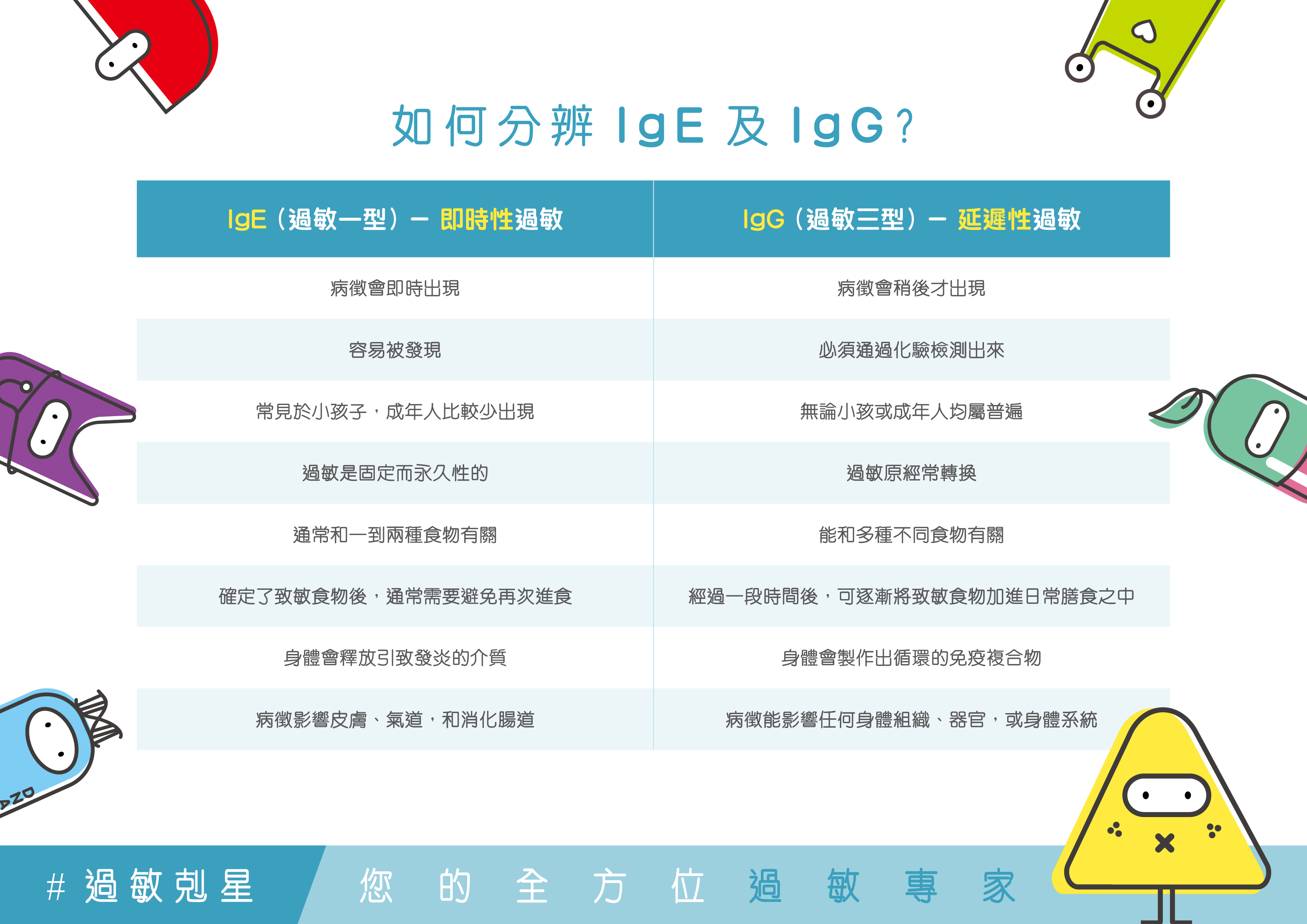# ApexHealth IgG+ 延迟性食物过敏测试-222

223項目

• ## 超过200项IgG+延迟性食物过敏测试

HK\$4,000.0

1 基本项目

• 青豆

• 绿髮藻
• 螺旋藻
• 海苔
• 鯷鱼
• 藤壶
• 鲈鱼
• 鱼子酱
• 蛤蜊
• 狮蚶 (赤贝 )
• 鳕鱼
• 螃蟹
• 墨鱼
• 鳗鱼 (鳝鱼 )
• 黑线鳕鱼
• 鳕鱼 (Hake)
• 龙虾
• 鲭鱼
• 鮟鱇鱼
• 青口
• 八爪鱼
• 河鲈鱼
• 梭子鱼
• 高眼鲽
• 蛏子
• 鮭鱼 (叁文鱼 )
• 沙甸鱼
• 扇贝
• 鲷鱼
• 红鲷鱼
• 龙脷鱼
• 鱿鱼
• 鳟鱼
• 吞拿鱼
• 鲽鱼 (多宝鱼 )

• 鲤鱼
• 鲱鱼 (希灵鱼 )
• 剑鱼

• 蛋白
• 蛋黄
• 水牛奶
• 牛奶
• 山羊奶
• 绵羊奶

• 大麦
• 古斯米
• 杜兰小麦
• 麦胶蛋白
• 麦芽
• 燕麦
• 黑麦
• 斯佩尔特小麦
• 小麦

• 米糠
• 苋穀
• 荞麦
• 玉米
• 粗玉米粉
• 稻米
• 木薯粉

• 李子（梅子）
• 红石榴
• 葡萄乾
• 山莓
• 红醋栗（红加仑子）
• 大黄
• 草莓（士多啤梨）
• 西瓜
• 苹果
• 牛油果
• 香蕉
• 黑莓
• 黑加仑子
• 樱桃（车厘子）
• 蔓越莓（小红莓）
• 无花果
• 葡萄
• 西柚
• 番石榴
• 奇异果
• 柠檬
• 莱檬（青柠）
• 荔枝
• 芒果
• 蜜瓜
• 桑椹
• 桃驳李（油桃）
• 橄榄
• 木瓜
• 蜜桃
• 凤梨（菠萝）

• 菜蓟（亚枝竹）
• 芦笋
• 茄子
• 蚕豆
• 绿豆
• 红腰豆
• 白芸豆
• 红菜头
• 西兰花
• 抱子甘蓝
• 红捲心菜
• 捲心菜（椰菜）
• 酸豆
• 胡萝蔔
• 椰菜花
• 芹菜
• 莙薘菜（猪菜）
• 鹰嘴豆
• 菊苣根
• 黄瓜
• 茴香菜（香丝菜）
• 韭葱（扁葱 / 京葱）
• 扁豆
• 生菜（唐生菜）
• 菜葫芦
• 洋葱
• 椒（混合）
• 薯仔
• 藜麦
• 白萝蔔
• 火箭菜
• 薤白
• 黄豆
• 菠菜
• 南瓜
• 蕃薯（地瓜）
• 蕃茄
• 芜菁（圆菜头）
• 豆瓣菜（西洋菜）
• 木薯

• 牛肉
• 鸡肉
• 鸭肉
• 山羊肉
• 马肉
• 绵羊肉
• 鸵鸟肉
• 水牛肉
• 鹧鸪
• 猪肉
• 鹌鹑
• 兔肉
• 火鸡肉
• 小牛肉
• 鹿肉
• 野猪肉

• 罗勒 (九层塔 )
• 月桂叶
• 洋金菊
• 辣椒
• 红辣椒
• 肉桂
• 丁香
• 芫荽
• 孜然 (阿拉伯茴香 )
• 咖哩
• 莳萝 (洋茴香 )
• 大蒜
• 银杏
• 人蔘
• 啤酒花
• 甘草
• 墨角兰
• 薄荷 (Mint)
• 芥菜籽
• 肉荳蔻
• 香芹
• 黑 / 白胡椒
• 薄荷
• 迷迭香
• 藏红花
• 鼠尾草
• 龙蒿
• 麝香草
• 香草 (云呢拿 )

• 开心果
• 油菜籽
• 芝麻
• 葵花籽
• 油莎草 (铁荸荠 )
• 核桃
• 杏仁
• 巴西坚果
• 腰果
• 椰子
• 亚麻籽
• 榛子
• 花生
• 松子

• α- 乳清蛋白
• β-乳球蛋白（牛奶成分）
• 酪蛋白（牛奶成分）

• 蓝莓

• 八角
• 夏威夷果仁

• 小米

• 蕁麻

• 琼脂 (大菜丝 )
• 芦荟
• 蔗糖
• 长角豆
• 栗子
• 可可豆
• 咖啡豆
• 可乐果
• 蜜糖
• 蘑菇
• 红茶
• 绿茶
• 食物添加剂
• 麵包酵母
• 啤酒酵母

### 报告

• 医生讲解报告

• IgE 综合过敏测试

涵盖吸入性及食物型致敏原项目多达71种，透过进行IgE即时性过敏测试，能够检测出您对食物和环境致敏原的免疫球蛋白E的浓度，从而知道哪些致敏原导致您有较严重的过敏反应，能了解及改善自己的过敏状况。医生讲解报告。

40% off

HK\$1,608.0
HK\$2,680

ApexHealth的延迟性食物过敏测试拥有超过222种食物检测，是香港目前能检测最多食物种类的延迟性食物过敏测试。

7个工作天完成食物致敏原分析。• 客户收到由「健康网购health.ESDlife」寄出之确认成功付款电邮后，ApexHealth 将于1-2个工作天内，致电客户预约身体检查的时间及地点。客户必须于预约当天出示身份证及打印订购确认信以确认身份。
• 本身体检查计划有效期为2个月，客户必须于2个月内(由确认付款日期起计)接受有关检查，逾期作废。
• 进行健康检查後，一般情况下，需大概2星期跟进检查报告。
• 订购一经确认，不设更改已订购的计划，转让给第三者及／或退款。
• 如有任何争议，「健康网购health.ESDlife」及 ApexHealth 保留最终决定权。

• 所有健康检查/服务并非作为医务诊断或治疗用途。当阁下身体健康出现任何疾病征兆时，应立即咨询有认可资格的医生，作出诊断及治疗。
• 本服务/产品由商户提供。生活易【健康网购 health.ESDlife】并没有经营或提供本服务/产品。有关此服务/产品的错漏或延误，或因使用此服务/产品而引致的损失、损害、受伤或法律诉讼，健康网购health.ESDlife概不负责。一切有关的索偿或查询，须向提供服务之体检中心或商户提出。